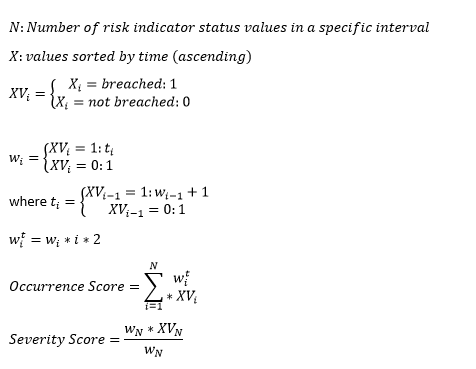# How is Occurrence Score of a KRI calculated

1. Take the risk indicator status (breached/not breached) of a site over a period of time in specific intervals.
2. Order them from earliest to latest
3. Iterate over each collected status (from second earliest to latest) and assign a weight to each value:
1.  If the current status is "not breached" then assign a weight of 1
2. If the current status is "breached" check the previous value
3. If the previous status is "not breached", assign a weight of 1
4. If the previous status is "breached", increase the weight of the previous value by one
4. Optionally transform the weights to increase effect each weight has.
5. Multiply each status with its weight (where "breached" is 1 and "not breached" is 0) and calculate the sum of the products.
6. Divide the latest value by the sum of all weights.
7. This value now is the occurrence score of a risk indicator on site level for a specific site.

Mathematical explanation